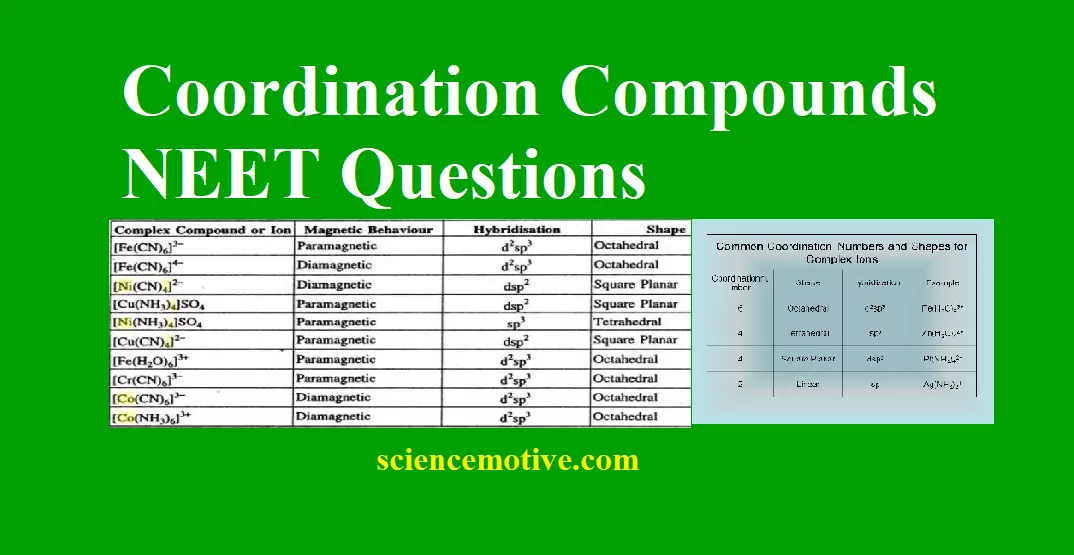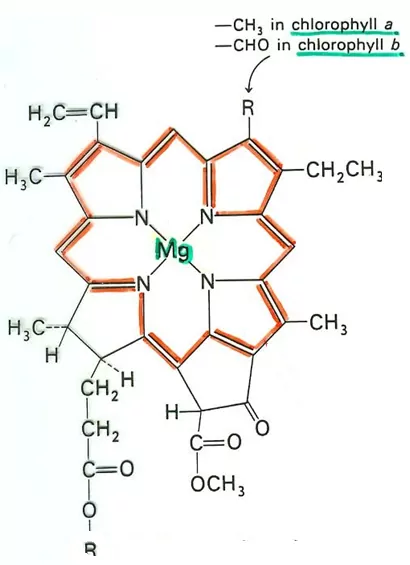# Coordination Compounds NEET QuestionsCoordination Compounds NEET Questions

Que 1. In K4[Fe(CN)6]
(a) (CN) are linked with primary valency
(b) (CN) are linked with secondary valency
(c) K is linked with secondary valency
(d) K is linked with non-ionic valency
Ans 1. (b) (CN) are linked with secondary valency
Explanation: (CN) are linked with secondary valency to the central Fe metal ion. Ligand (CN) is linked to the central atom via a dative bond.

Que 2. In the coordination compound, K4[Ni(CN)4], the oxidation state of nickel is
(a) 0                                                                        (b) +1
(c) +2                                                                      (d) –1
Ans 2. (a) 0
Explanation: Let oxidation state of Nickel be x
(1×4)+x+(4×−1)=0
⇒ 4+x−4=0
⇒ x=0

Que 3. The co-ordination number of copper in cuprammonium sulphate is
(a) 2                                                                                        (b) 6
(c) 4                                                                                        (d) – 4
Ans 3. (c) 4

Que 4. Which of the following acts as a bidentate ligand in complex formation
(a) Acetate                                                                              (b) Oxalate
(c) Thiocyanate                                                                      (d) EDTA
Ans 4. (b) Oxalate (C2O42-)

Que 5. [EDTA]4– is a:
(a) monodentate ligand
(b) bidentate ligand
Structure:Coordination Compounds NEET Questions

Que 6. The co-ordination number of cobalt in the complex [Co(en)2Br2]Cl2 is
(a) 2                                                                                        (b) 6
(c) 5                                                                                        (d) 4
Ans 6. (b) 6
Explanation:
In the given complex,
Co binds with two a bi-dentate ligand en (2 × 2 = 4) and two mono-dentate ligands Cl (2 × 1 = 2).
Therefore, its co-ordination number is 4 + 2 = 6.
In the given complex, Co binds with two a bi-dentate ligand en (2 × 2 = 4) and two mono-dentate ligands Cl (2 × 1 = 2).
Therefore, the co-ordination number is 4 + 2 = 6.

Que 7. The stabilization of coordination compounds due to chelation is called the chelate effect. Which of the following is the most stable complex species?
(a) [Fe(CO)5]                                                                       (b) [Fe(CN)6]3–
(c) [Fe(C2O4)3]3+                                                                (d) [Fe(H2O)6]3+
Ans 7. (c) [Fe(C2O4)3]3+
Explanation: [Fe(C2O4)3]3 is a coordination compound that contains oxalate ions as a chelating ligand. Hence, it stabilizes the coordination compound by chelating the Fe3+ ion.

Que 8. Which of the following ligands forms a chelate
(a) Acetate                                                                              (b) Oxalate
(c) Cyanide                                                                             (d) Ammonia
Ans 8. (b) Oxalate (C2O42-)

Que 9. Which of the following is a complex metal other than transition metal?
(a) Haemoglobin                                                                     (b) Chlorophyll
(c) Ferrocene                                                                           (d) Vitamin B12
Ans 9. (b) Chlorophyll
Explanation:Coordination Compounds NEET Questions

Que 10. According to Werner’s theory
(a) Primary valency can be ionized
(b) Secondary valency can be ionized
(c) Primary and secondary valencies both cannot be ionized
(d) Only primary valency cannot be ionized
Ans 10. (a) Primary valency can be ionized

Que 11. Which of the following is not true for ligand-metal complex
(a) Larger the ligand, the more stable is the metal-ligand bond
(b) Highly charged ligand forms strong bond
(c) Larger the permanent dipole moment of the ligand, the more stable is the bond
(d) Greater the ionization potential of central metal, the stronger is the bond
Ans 11. (b) Highly charged ligand forms a strong bond

Que 12. Pentaamminenitrocobalt (III) cation possesses the property of:
(a) Physisorption
(b) Chirality
(d) Versatile reducing agent
Ans 12. (d) Versatile reducing agent
In the case of nitrito ion (NO2), either nitrogen or an oxygen atom may act as a donor giving two different linkage isomers.Coordination Compounds NEET Questions

Que 13. The anion [Co(C2O4)3]3– involves which type of hybridization?
(a) d2sp3                                                                                  (b) sp3d2
(c) d3sp2                                                                                  (d) sp2d3
Ans 13. (a)
Explanation: [Co(C2​O4​)3​]3−
= CO3+ = [Ar]3d6
C2​O42− ​= Strong field ligand.
= d2sp3 Hybridisation.
= Octahedral.
(C2​O4​)2− = Bidentate Ligand

Que. 14. Which of the following is not optically active?
(a) [Co(en)3]3+                                                                        (b) [Cr(ox)3]3-
(c) cis-[CoCl2(en)2]+                                                               (d) trans-[CoCl2(en)2]+
Ans 14. (d) trans-[CoCl2(en)2]+
Explanation: It is because square planar and octahedral complexes exhibit geometrical isomerism (cis- and trans-forms) and cis- form is only optically active.

Que 15. Indicate the complexion which shows geometrical isomerism.
(a) [Cr(H2O)4Cl2]+                                                                  (b) [Pt(NH3)3Cl]
(c) [Pt(NH3)6]3+                                                                       (d) [CO(CN)5(NC)]3–
Ans 15. (a) [Cr(H2O)4Cl2]+
Explanation: It is because square planar and octahedral complexes exhibit geometrical isomerism.

Coordination Compounds NEET Questions